• 最优解存在的条件 在凸优化问题中应用Weierstrass 定理： 引理1：如果凸优化问题的可行域为非空紧集，且在上连续，则最优解一定存在。 引理2：已知函数连续，且其定义域为开集，那么其下水平集为紧集，当且仅当为...
Weierstrass 定理
令为的非空子集，在的所有点处下半连续。假设下列三个条件之一成立:
(1)是紧集；
(2)是闭集且是强制的；
(3)存在一个标量，使得截集为非空紧集。 那么，在上的最小值点的集合为非空紧集。
最优解存在的条件
在凸优化问题中应用Weierstrass 定理：
引理1：如果凸优化问题的可行域为非空紧集，且在上连续，则最优解一定存在。
引理2：已知函数连续，且其定义域为开集，那么其下水平集为紧集，当且仅当为强制函数。
引理3：如果可行域(无约束优化问题)，连续且是强制函数，则最优解一定存在。
引理4：如果可行域为非空闭集，且是强制函数，则最优解一定存在。
最优解唯一的条件
如果目标函数是严格凸的，可行域是凸集，则最优解唯一。
当目标函数为非常数的线性函数时(但)，如果最优解存在，则一定属于可行域的边界点。
当目标函数为非常数的线性函数，并且可行域是严格凸的、全为的闭集，如果最优解存在，则唯一。

展开全文• 本文讨论了一类多目标规划问题，得到了最优解存在和唯一的充分条件
• // 添加筛选条件 String selection = MediaStore.Images.Media.DISPLAY_NAME + "=" + "'" + saveFileName + "'"; Cursor mCursor = contentResolver.query(mImageUri, projection, selection, null, null); ...
安卓Q中google彻底禁止了第三发应用获取imei，并且mac地址返回的地址也变成了02:00:00:00:00:00。
这是官方给出的解决方案：
https://developer.android.com/training/articles/user-data-ids
这是官网的截图：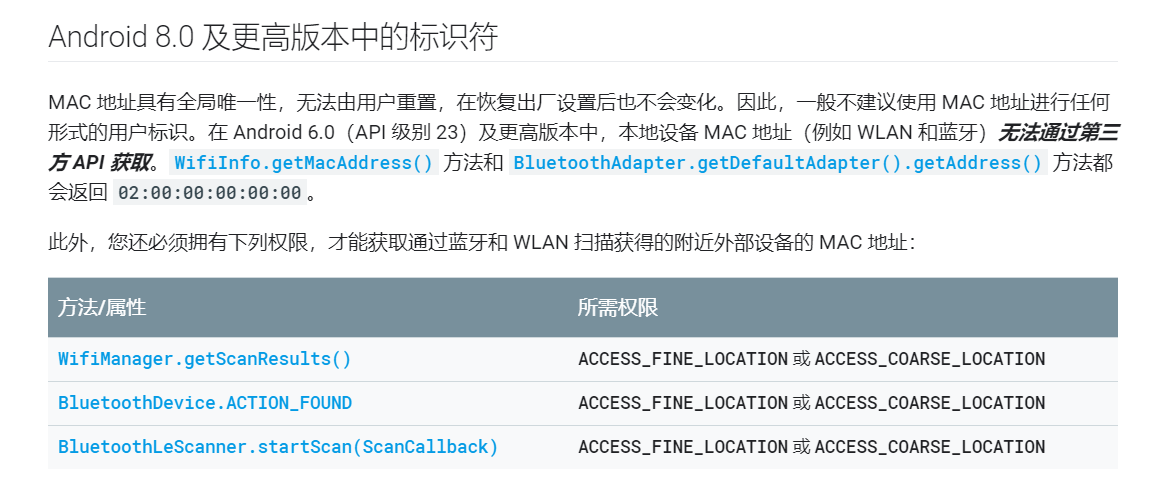方法一:
既然这两个都没法获取了，所以想到获取ANDROID_ID：
    public static String getAndroidId (Context context) {
String ANDROID_ID = Settings.System.getString(context.getContentResolver(), Settings.System.ANDROID_ID);
return ANDROID_ID;
}

但是发现不同应用的正式包在相同设备上得到的ANDROID_ID结果不同，因此显然该方案不可行。
方法二: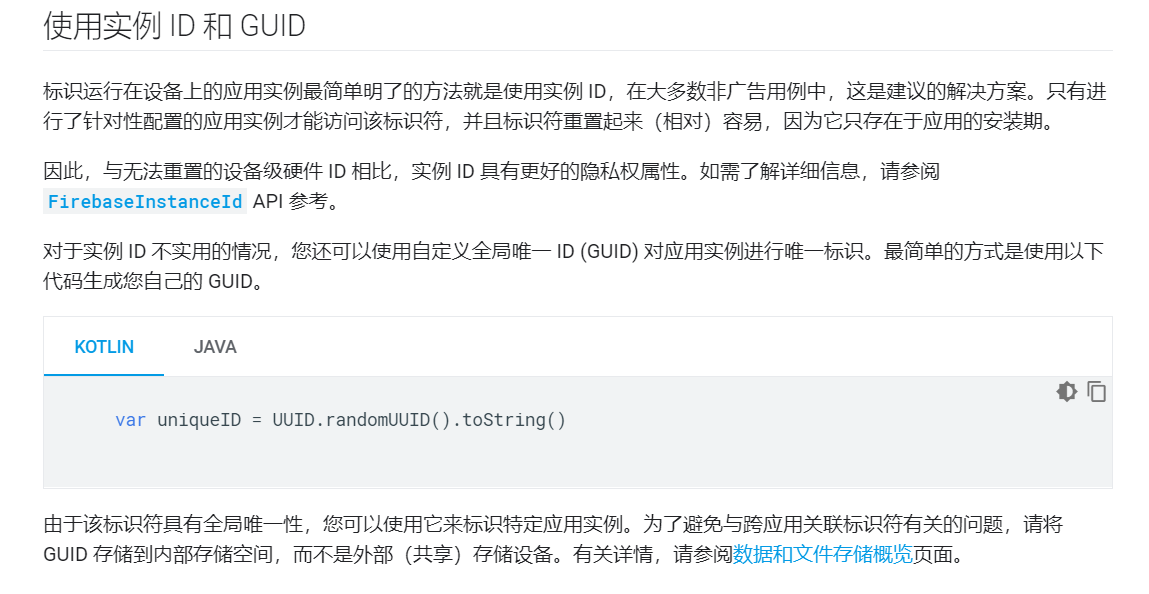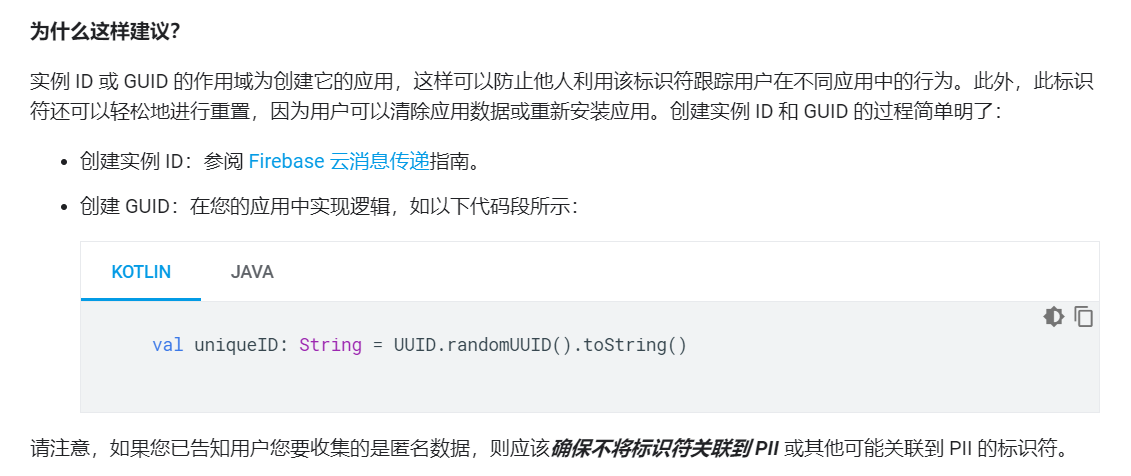好的，那么就使用UUID：
String uuidStr = UUID.randomUUID().toString();
将UUID以文件的形式保存在多媒体文件目录下，这样一来各个不同的应用间都能采用同一个UUID。生成UUID。其中 saveFileName 为存放uuid数据的文件名：

/**
* 在媒体文件中 生成fileName文件
* 向Mediastore添加内容
*
* @param saveFileName 保存文件的名称
*/
private void creatUUIDFile(String saveFileName) {
ContentValues values = new ContentValues();
values.put(MediaStore.Images.Media.DISPLAY_NAME, saveFileName);
values.put(MediaStore.Images.Media.MIME_TYPE, "image/*");
// TODO: 2019-10-11 IS_PENDING = 1表示对应的item还没准备好
values.put(MediaStore.Images.Media.IS_PENDING, 1);

ContentResolver resolver = this.getContentResolver();
Uri collection = MediaStore.Images.Media.getContentUri(MediaStore.VOLUME_EXTERNAL_PRIMARY);

Uri uri = resolver.insert(collection, values);

try {
//访问 对于单个媒体文件，请使用 openFileDescriptor()。
ParcelFileDescriptor fielDescriptor = resolver.openFileDescriptor(uri, "w", null);
FileOutputStream outputStream = new FileOutputStream(fielDescriptor.getFileDescriptor());
try {
//讲UUID写入到文件中
String uuidStr = UUID.randomUUID().toString();
outputStream.write(uuidStr.getBytes());
outputStream.close();
Log.d(TAG, "写入 uuidStr:" + uuidStr);
} catch (IOException e) {
e.printStackTrace();
}
values.clear();
values.put(MediaStore.Images.Media.IS_PENDING, 0);          //设置为0
resolver.update(uri, values, null, null);
} catch (FileNotFoundException e) {
e.printStackTrace();
}
}
如果需要判断是否已经存在存放uuid的文件，则用下面的方法：
    /**
* 检查文件是否存在
*
* @param saveFileName 保存文件的名称
* @return true 为存在   false为不存在
*/
private boolean checkUUIDFileByUri(String saveFileName) {
Uri mImageUri = MediaStore.Images.Media.EXTERNAL_CONTENT_URI;
String[] projection = {
MediaStore.Images.Media.DISPLAY_NAME,
MediaStore.Images.Media._ID
};
//查询
ContentResolver contentResolver = this.getContentResolver();

// 添加筛选条件
String selection = MediaStore.Images.Media.DISPLAY_NAME + "=" + "'" + saveFileName + "'";
Cursor mCursor = contentResolver.query(mImageUri, projection, selection, null, null);

String getSaveContent = "";
if (mCursor != null) {
while (mCursor.moveToNext()) {

int fileIdIndex = mCursor.getColumnIndex(MediaStore.Images.Media._ID);
String thumbPath = MediaStore.Images.Media.EXTERNAL_CONTENT_URI.buildUpon()
.appendPath(String.valueOf(mCursor.getInt(fileIdIndex))).build().toString();
Uri fileUri = Uri.parse(thumbPath);
try {
ParcelFileDescriptor fielDescriptor = contentResolver.openFileDescriptor(fileUri, "r", null);
FileInputStream inputStream = new FileInputStream(fielDescriptor.getFileDescriptor());
getSaveContent = inputStreamToString(inputStream);

} catch (FileNotFoundException e) {
e.printStackTrace();
}

//只有在得到的唯一标识符不为空的情况下才结束循环，否则一直循环
if (!TextUtils.isEmpty(getSaveContent)) {
break;
}
}
mCursor.close();

}

return !getSaveContent.equals("");
}

上面代码中的inputStreamToString（）方法为流转字符串，代码如下：
    /**
* 流转为字符串
*
* @param is 流
* @return 转换完成的字符串
*/
public String inputStreamToString(InputStream is) {
StringBuilder sb = new StringBuilder();

String line;
try {
sb.append(line).append("/n");
}
} catch (IOException e) {
e.printStackTrace();
} finally {
try {
is.close();
} catch (IOException e) {
e.printStackTrace();
}
}
return sb.toString();
}

好的，先这样。
展开全文• 线性规划最优解集的数学理论与计算方法，彭岳林，，本文导出了线性规划最优解集的连续性、切向等值性、唯一性和法向严格单调性四大特征,建立了线性规划最优解集的一个充分必要条件,�
• 系数矩阵分析 、单纯形表、最优解判定


文章目录
一、

(

C

N

T

−

C

B

T

B

−

1

N

)

( C_N^T - C_B^T B^{-1}N )

系数 分析二、

C

B

C_B

X

B

X_B

分析三、

C

N

C_N

X

N

X_N

分析四、

B

−

1

N

B^{-1}N

分析五、单纯形表六、最优解判定

在上一篇博客 【运筹学】线性规划数学模型 ( 单纯形法 | 最优解判定原则 | 可行解表示 | 目标函数推导 | 目标函数最大值分析 ) 博客中讲解了最优解判定的推导过程 , 基本原理就是
将可行解

{

X

B

=

B

−

1

b

−

B

−

1

N

X

N

X

N

\begin{cases} X_B = B^{-1}b - B^{-1}NX_N \\ \\X_N \end{cases}

代入线性规划目标函数

m

a

x

Z

=

C

B

T

X

B

+

C

N

T

X

N

max Z = C_B^TX_B + C_N^TX_N

中 ,  最终得到一个

m

a

x

Z

=

b

0

+

(

C

N

T

−

C

B

T

B

−

1

N

)

X

N

maxZ = b_0 + ( C_N^T - C_B^T B^{-1}N )X_N

目标函数 ,  只有当

(

C

N

T

−

C

B

T

B

−

1

N

)

( C_N^T - C_B^T B^{-1}N )

系数小于等于

0

0

时 , 该目标函数才是最大值 , 该解是最优解 ;

单纯形法解线性规划的三大问题 : 查找初始基可行解 , 判定是否是最优解 , 如何迭代基可行解 , 当前讨论的问题是判定最优解 ;

一、

(

C

N

T

−

C

B

T

B

−

1

N

)

( C_N^T - C_B^T B^{-1}N )

系数 分析

目标函数推导 :

m

a

x

Z

=

C

B

T

(

B

−

1

b

−

B

−

1

N

X

N

)

+

C

N

T

X

N

=

C

B

T

B

−

1

b

−

C

B

T

B

−

1

N

X

N

+

C

N

T

X

N

=

b

0

+

(

C

N

T

−

C

B

T

B

−

1

N

)

X

N

=

b

0

+

σ

m

+

1

x

m

+

1

+

σ

m

+

2

x

m

+

2

+

⋯

+

σ

n

x

n

\begin{array}{lcl} max Z &=& C_B^T ( B^{-1}b - B^{-1}NX_N ) + C_N^TX_N \\\\ &=& C_B^T B^{-1}b - C_B^T B^{-1}NX_N + C_N^TX_N\\\\ &=& b_0 + ( C_N^T - C_B^T B^{-1}N )X_N \\\\ &=& b_0 + \sigma_{m+1} x_{m+1} + \sigma_{m+2} x_{m+2} + \cdots + \sigma_{n} x_{n} \\\\ \end{array}

上述分析到在

X

N

=

O

X_N = O

时 , 如果使目标函数取值最大 ,

σ

m

+

1

x

m

+

1

+

σ

m

+

2

x

m

+

2

+

⋯

+

σ

n

x

n

\sigma_{m+1} x_{m+1} + \sigma_{m+2} x_{m+2} + \cdots + \sigma_{n} x_{n}

系数都小于等于

0

0

时 , 满足上述要求 ;

上面的系数是通过

(

C

N

T

−

C

B

T

B

−

1

N

)

( C_N^T - C_B^T B^{-1}N )

计算出来的 ;

C

N

T

C_N^T

是目标函数中 , 非基变量的系数 ;

C

B

T

C_B^T

是目标函数中 , 基变量的系数 ;

B

−

1

N

B^{-1}N

:

B

−

1

N

B^{-1}N

是将基矩阵进行变换 , 将基矩阵变换为单位阵

I

I

, 非基矩阵就是

B

−

1

N

B^{-1}N

;

二、

C

B

C_B

X

B

X_B

分析

C

B

C_B

与

X

B

X_B

矩阵分析 :

C

B

T

C_B^T

矩阵与

X

B

X_B

的对应关系 ,

X

B

=

(

x

1

x

2

⋮

x

m

)

X_B =\begin{pmatrix} x_{1} \\ x_{2} \\ \vdots\\ x_m \end{pmatrix}

,

C

B

=

(

c

1

c

2

⋮

c

m

)

C_B =\begin{pmatrix} c_{1} \\ c_{2} \\ \vdots\\ c_m \end{pmatrix}

,

C

B

T

=

(

c

1

c

2

⋯

c

m

)

;

目标函数为

m

a

x

Z

=

C

B

T

X

B

+

C

N

T

X

N

max Z = C_B^T X_B+ C_N^TX_N

, 在目标函数中 , 有以下对应关系 :

其中的

C

B

T

X

B

=

(

c

1

c

2

⋯

c

m

)

×

(

x

1

x

2

⋮

x

m

)

C_B^T X_B = \begin{pmatrix} c_{1} \quad c_{2} \quad \cdots \quad c_m \end{pmatrix} \times \begin{pmatrix} x_{1} \\ x_{2} \\ \vdots\\ x_m \end{pmatrix}

,

c

1

c_1

与

x

1

x_1

对应 ,

c

m

c_m

与

x

m

x_m

对应 ;

三、

C

N

C_N

X

N

X_N

分析

C

N

C_N

与

X

N

X_N

矩阵分析 :

C

N

T

C_N^T

矩阵与

X

N

X_N

的对应关系 ,

X

N

=

(

x

m

+

1

x

m

+

2

⋮

x

n

)

X_N =\begin{pmatrix} x_{m+1} \\ x_{m+2} \\ \vdots\\ x_n \end{pmatrix}

,

C

N

=

(

c

m

+

1

c

m

+

2

⋮

c

n

)

C_N =\begin{pmatrix} c_{m+1} \\ c_{m+2} \\ \vdots\\ c_n \end{pmatrix}

,

C

N

T

=

(

c

m

+

1

c

m

+

2

⋯

c

n

)

;

目标函数为

m

a

x

Z

=

C

B

T

X

B

+

C

N

T

X

N

max Z = C_B^T X_B+ C_N^TX_N

, 在目标函数中 , 有以下对应关系 :

同理 ,

C

N

C_N

与

X

N

X_N

矩阵计算 ,

C

N

T

X

N

=

(

c

m

+

1

c

m

+

2

⋯

c

n

)

×

(

x

m

+

1

x

m

+

2

⋮

x

n

)

C_N^T X_N = \begin{pmatrix} c_{m+1} \quad c_{m+2} \quad \cdots \quad c_n \end{pmatrix} \times \begin{pmatrix} x_{m+1} \\ x_{m+2} \\ \vdots\\ x_n \end{pmatrix}

,

c

m

+

1

c_{m+1}

与

x

m

+

1

x_{m+1}

对应 ,

c

n

c_n

与

x

n

x_n

对应 ;

四、

B

−

1

N

B^{-1}N

分析

B

−

1

N

B^{-1}N

分析 :
线性规划约束条件是

B

X

B

+

N

X

N

=

b

BX_B + NX_N = b

, 其系数矩阵是

(

B

N

)

\begin{pmatrix} \, B \quad N \, \end{pmatrix}

样式的 , 在

B

X

B

+

N

X

N

=

b

BX_B + NX_N = b

两端都乘以

B

−

1

B^{-1}

, 然后移项得到

X

B

+

B

−

1

N

X

N

=

B

−

1

b

X_B + B^{-1}NX_N= B^{-1}b

, 此时当基变量是单位阵

I

I

时 , 非基变量就是

B

−

1

N

B^{-1}N

, 系数矩阵是

(

I

B

−

1

N

)

\begin{pmatrix} \, I \quad B^{-1}N \, \end{pmatrix}

五、单纯形表

通过计算

(

C

N

T

−

C

B

T

B

−

1

N

)

( C_N^T - C_B^T B^{-1}N )

, 是否是负数 , 可以判定当前的解是否是最优解 ;

将上述

C

N

T

C_N^T

,

C

B

T

C_B^T

,

B

−

1

B^{-1}

,

N

N

等写在一个表中 , 该表就是如下单纯形表 ;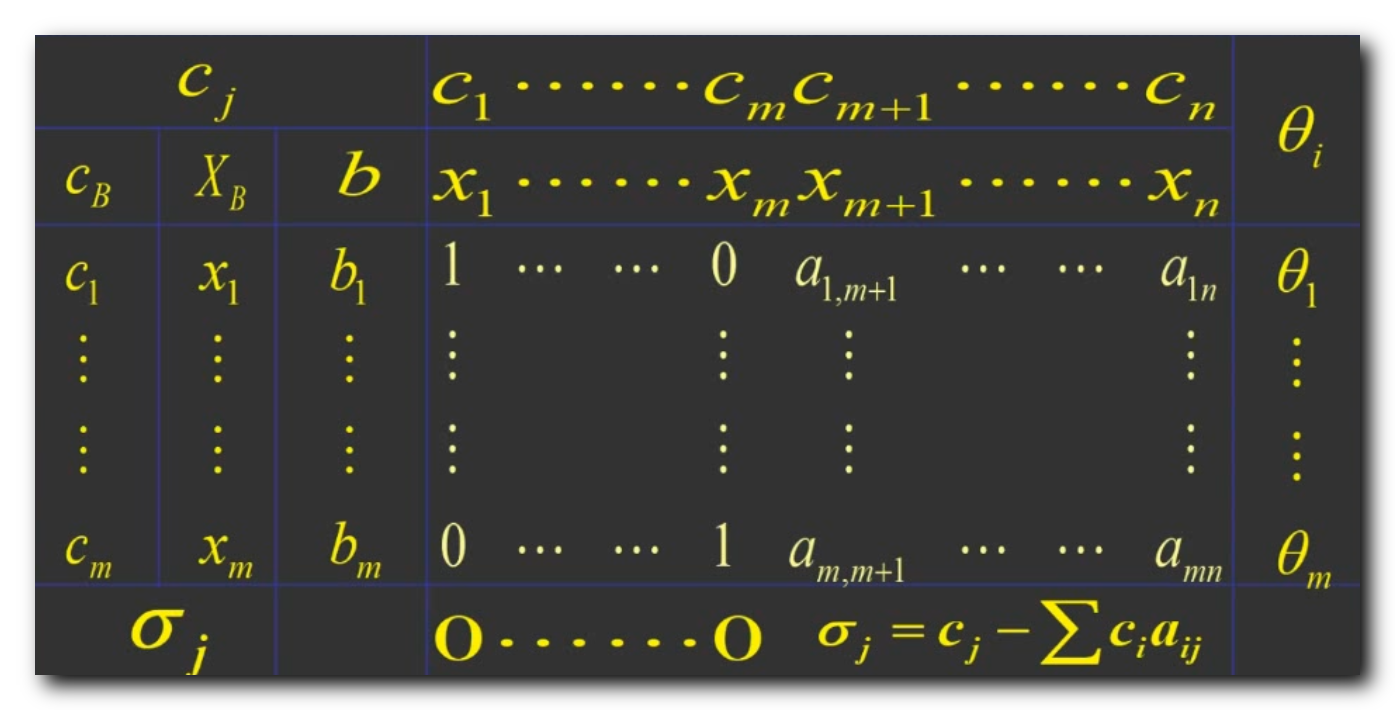在上述单纯形表中 , 假设前

m

m

个向量是基变量 , 将基变量对应的矩阵 , 变换为单位阵 , 单位阵

I

I

与

B

−

1

N

B^{-1}N

如下图所示 :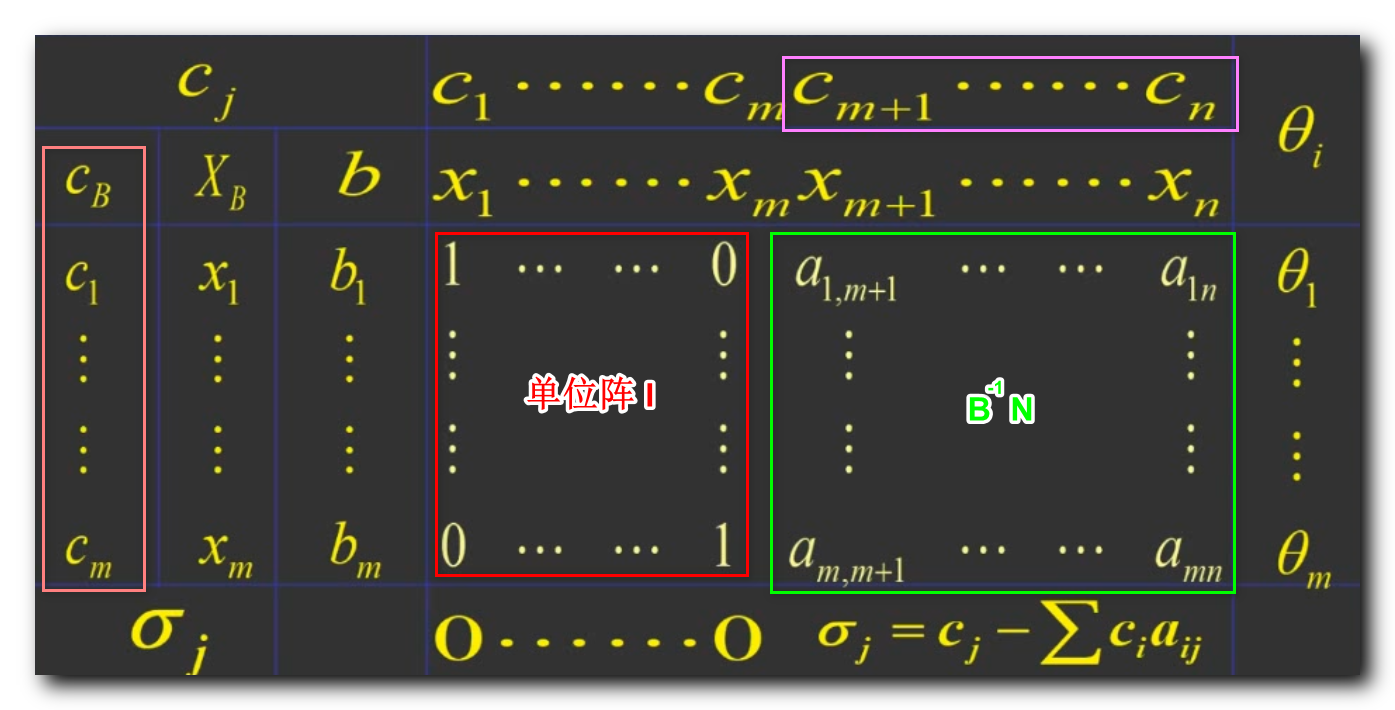六、最优解判定

目标函数推导 :

m

a

x

Z

=

C

B

T

(

B

−

1

b

−

B

−

1

N

X

N

)

+

C

N

T

X

N

=

C

B

T

B

−

1

b

−

C

B

T

B

−

1

N

X

N

+

C

N

T

X

N

=

b

0

+

(

C

N

T

−

C

B

T

B

−

1

N

)

X

N

=

b

0

+

σ

m

+

1

x

m

+

1

+

σ

m

+

2

x

m

+

2

+

⋯

+

σ

n

x

n

\begin{array}{lcl} max Z &=& C_B^T ( B^{-1}b - B^{-1}NX_N ) + C_N^TX_N \\\\ &=& C_B^T B^{-1}b - C_B^T B^{-1}NX_N + C_N^TX_N\\\\ &=& b_0 + ( C_N^T - C_B^T B^{-1}N )X_N \\\\ &=& b_0 + \sigma_{m+1} x_{m+1} + \sigma_{m+2} x_{m+2} + \cdots + \sigma_{n} x_{n} \\\\ \end{array}

最终目标是计算

σ

m

+

1

,

σ

m

+

2

,

⋯

,

σ

n

\sigma_{m+1} , \sigma_{m+2} , \cdots , \sigma_{n}

系数是否小于等于

0

0

;

上面已经推导出目标函数的系数是

(

C

N

T

−

C

B

T

B

−

1

N

)

( C_N^T - C_B^T B^{-1}N )

矩阵 :

C

N

T

=

(

c

m

+

1

c

m

+

2

⋯

c

n

)

C

B

T

=

(

c

1

c

2

⋯

c

m

)

B

−

1

N

=

[

a

1

,

m

+

1

⋯

a

1

n

⋮

⋮

⋮

a

m

,

m

+

1

⋯

a

m

n

]

B^{-1}N =\begin{bmatrix} &a_{1,m+1} & \cdots & a_{1n} & \\\\ &\vdots & \vdots & \vdots & \\\\ &a_{m,m+1} & \cdots & a_{mn} & \end{bmatrix}

(

C

N

T

−

C

B

T

B

−

1

N

)

=

(

c

m

+

1

c

m

+

2

⋯

c

n

)

−

(

c

1

c

2

⋯

c

m

)

×

[

a

1

,

m

+

1

⋯

a

1

n

⋮

⋮

⋮

a

m

,

m

+

1

⋯

a

m

n

]

( C_N^T - C_B^T B^{-1}N ) = \begin{pmatrix} c_{m+1} \quad c_{m+2} \quad \cdots \quad c_n \end{pmatrix} - \begin{pmatrix} c_{1} \quad c_{2} \quad \cdots \quad c_m \end{pmatrix} \times \begin{bmatrix} &a_{1,m+1} & \cdots & a_{1n} & \\\\ &\vdots & \vdots & \vdots & \\\\ &a_{m,m+1} & \cdots & a_{mn} & \end{bmatrix}

=

(

σ

m

+

1

σ

m

+

2

⋯

σ

n

)

σ

j

\sigma_j

个系数的公式如下 :

σ

j

=

c

j

−

∑

c

i

a

i

j

\sigma_j = c_j - \sum c_i a_{ij}

其中

c

j

c_j

对应的是非基变量在目标函数系数 ,

c

i

c_i

是基变量在目标函数中的系数 ,

a

i

j

a_{ij}

是

B

−

1

N

B^{-1}N

中的矩阵向量 , 代表一列 ;

如 :

σ

m

+

1

=

c

m

+

1

−

∑

i

=

1

n

c

i

a

i

,

m

+

1

=

c

m

+

1

−

c

1

a

1

,

m

+

1

−

c

2

a

2

,

m

+

1

−

⋯

−

c

n

a

n

,

m

+

1

\begin{array}{lcl} \sigma_{m+1} &=& c_{m+1} - \sum_{i = 1}^{n} c_i a_{i, m+1} \\\\ &=& c_{m+1} - c_1a_{1,m+1} - c_2a_{2,m+1} - \cdots - c_na_{n,m+1} \end{array}

如果所有的

σ

j

\sigma_j

系数值, 都小于等于

0

0

, 说明该基可行解就是最优解 ;

最优解判定示例 :

① 不是最优解的情况 : 如果最终计算的系数是

m

a

x

Z

=

88

+

3

x

6

−

4

x

7

max Z = 88 + 3x_6 - 4x_7

, 此时

x

6

x_6

的系数

σ

6

\sigma_6

大于

0

0

, 该函数不是最优解 ;
② 是最优解的情况 : 如果最终计算的系数是

m

a

x

Z

=

88

−

3

x

6

−

4

x

7

max Z = 88 - 3x_6 - 4x_7

, 所有的系数都是小于等于

0

0

的值 , 该基矩阵对应的解就是最优解 ;
只要有一个系数不是小于等于

0

0

的 , 那么该解就不是最优解 , 就需要继续向下迭代 ;
展开全文线性规划 单纯形表
• 一、最优解判别、 二、初始基可行解、 三、运费修改可行性方案、 四、闭回路法


文章目录
一、最优解判别二、初始基可行解三、运费修改可行性方案四、闭回路法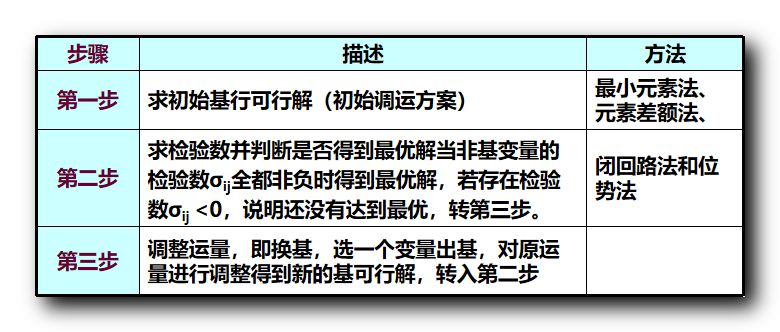一、最优解判别

在上两篇博客 【运筹学】表上作业法 ( 求初始基可行解 | 最小元素法 ) , 【运筹学】表上作业法 ( 最小元素法分析 | Vogel 方法 ) 中 , 分别给出了表上作业法如何找初始基可行解 , 两种方法 " 最小元素法 " 和 " Vogel 方法 ( 差额法 ) " , 其中 Vogel 方法 得到的初始基可行解更靠近最优解 ;

下面开始判断该 初始基可行解 是否是 最优解 ;

最优解判别 : 得到一组 基可行解 之后 , 使用 检验数 判定该解是否是最优解 ;
检验数符号 : 变量

x

i

j

\rm x_{ij}

的检验数记作

λ

i

j

\rm \lambda_{ij}

;
检验数判定原则 : 运输规划的 目标函数求最小值 时 , 所有的 非基变量检验数

λ

i

j

\rm \lambda_{ij}

都非负 , 该基可行解就是最优解 , 该运输方案是最优方案 ;

求检验数的方法 : ① 闭回路法 , ② 位势法 ;

二、初始基可行解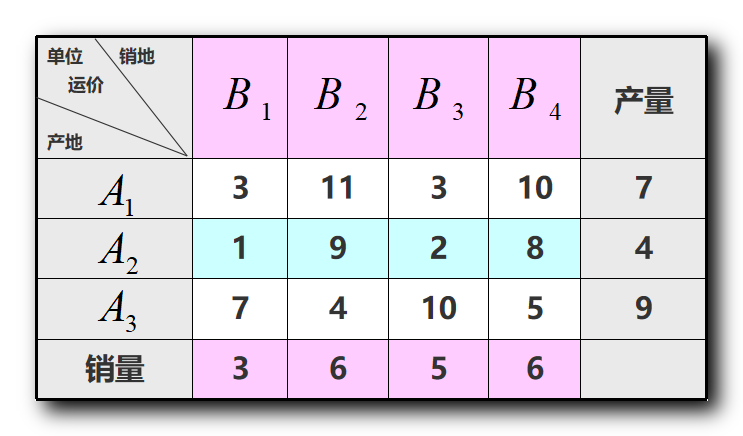使用最小元素法求得的初始基可行解 :

B

1

\rm B_1

B

2

\rm B_2

B

3

\rm B_3

B

4

\rm B_4

产量

A

1

\rm A_1

3

3

11

11

3

3

,

4

4

10

10

,

3

3

7

7

A

2

\rm A_2

1

1

,

3

3

9

9

2

2

,

1

1

8

8

4

4

A

3

\rm A_3

7

7

4

4

,

6

6

10

10

5

5

,

3

3

9

9

销量

3

3

6

6

5

5

6

6

使用 最小元素法, 得到初始基可行解 :

{

x

13

=

4

x

14

=

3

x

21

=

3

x

23

=

1

x

32

=

6

x

34

=

3

\begin{cases} \rm x_{13} = 4 \\\\ \rm x_{14} = 3 \\\\ \rm x_{21} = 3 \\\\ \rm x_{23} = 1 \\\\ \rm x_{32} = 6 \\\\ \rm x_{34} = 3 \end{cases}

使用 Vogel 方法求得初始基可行解 :

B

1

\rm B_1

B

2

\rm B_2

B

3

\rm B_3

B

4

\rm B_4

产量行差额

A

1

\rm A_1

3

3

,

2

2

1̸1

\not 11

3

3

,

5

5

1̸0

\not 10

7

7

0

0

A

2

\rm A_2

1̸

\not 1

,

1

1

9̸

\not 9

2̸

\not 2

8̸

\not 8

,

3

3

4

4

1̸

\not 1

A

3

\rm A_3

7̸

\not 7

4̸

\not 4

,

6

6

1̸0

\not 10

5̸

\not 5

,

3

3

9

9

2̸

\not 2

销量

3

3

6

6

5

5

6

6

列差额

2

2

5̸

\not 5

1

1

2̸

\not 2

使用 Vogel 方法, 得到初始基可行解 :

{

x

11

=

2

x

13

=

5

x

21

=

1

x

24

=

3

x

32

=

6

x

34

=

3

\begin{cases} \rm x_{11} = 2 \\\\ \rm x_{13} = 5 \\\\ \rm x_{21} = 1 \\\\ \rm x_{24} = 3 \\\\ \rm x_{32} = 6 \\\\ \rm x_{34} = 3 \end{cases}

推荐使用 Vogel 方法计算初始基可行解 ;

三、运费修改可行性方案

以最小元素法获得的初始基可行解为例 :

B

1

\rm B_1

B

2

\rm B_2

B

3

\rm B_3

B

4

\rm B_4

产量

A

1

\rm A_1

3

3

11

11

3

3

,

4

4

10

10

,

3

3

7

7

A

2

\rm A_2

1

1

,

3

3

9

9

2

2

,

1

1

8

8

4

4

A

3

\rm A_3

7

7

4

4

,

6

6

10

10

5

5

,

3

3

9

9

销量

3

3

6

6

5

5

6

6

当前的初始基可行解的总运费计算如下 :

(

3

×

4

)

+

(

10

×

3

)

+

(

1

×

3

)

+

(

2

×

1

)

+

(

4

×

6

)

+

(

3

×

5

)

=

86

\rm ( 3 \times 4 ) + ( 10 \times 3 ) + ( 1 \times 3 ) + ( 2 \times 1 ) + ( 4 \times 6 ) + ( 3 \times 5 ) = 86

提出问题 :
在上述运输规划表格中 ,

A

2

\rm A_2

没有向

B

4

\rm B_4

运输产品 , 如果想要增加该项的运输 ;

A

2

\rm A_2

的产量是固定的 , 不能凭空多出来 , 如果想要多给

B

4

\rm B_4

运输一部分 , 一定要减少其它销地的运输 ;
这里

A

2

\rm A_2

可以减少向

B

1

\rm B_1

或

B

3

\rm B_3

销地运输产品的个数 ;
假如想要减少

A

2

\rm A_2

产地运往

B

1

\rm B_1

销地的产品数量 , 但对于

B

1

\rm B_1

销地来说 , 从

A

2

\rm A_2

产地获取的产品少了 , 需要从其它产地获取更多产品 , 而此时其它的产地的产品运输都是饱和的 , 多不出来 ;

运量变化规则 :
单纯形法中每次迭代中 , 要选出一个 出基变量 , 和一个 入基变量 , 这两个成对出现 ;
同理在运输规划中 , 也有类似的概念 ; 增加某个方向的运量 , 需要立刻体现出 减少了某个方向的运量 , 增加一个 , 减少一个 ; 增加和减少交替出现 ;

不可行的修改方案 :
如果想要增加一个销地的运量 , 就需要减少另外一个销地的运量 , 但是注意 , 减少另外销地的运量不能影响其它的运输问题 , 上述情况下 增加

A

2

\rm A_2

向

B

4

\rm B_4

的运量 , 此时如果要 减少

A

2

\rm A_2

对

B

1

\rm B_1

的运量 , 会引起

B

1

\rm B_1

销地供货不足 , 导致另外的连锁反应 , 需要增加另外产地的向

B

1

\rm B_1

供货 , 但是

A

1

,

A

3

\rm A_1 , A_3

都没有可以增加供货的空间 ;
这样无法形成一个闭合回路 ;

可行的修改方案 :
增加

A

2

\rm A_2

向

B

4

\rm B_4

的运量 , 如果 减小

A

2

\rm A_2

向

B

3

\rm B_3

运输的产品数 ,

B

3

\rm B_3

得到的物资从

A

2

\rm A_2

减少 , 那么相应

A

1

\rm A_1

向

B

3

\rm B_3

供货需要增加 ,

A

2

\rm A_2

向

B

3

\rm B_3

的运输量最多减少

1

1

个 , 对于

A

1

\rm A_1

来说 , 向

A

3

\rm A_3

运输增加了 , 一定需要减少运往某个销地的运量 , 只能 减少

A

1

\rm A_1

到

B

4

\rm B_4

的运量 , 此时发现产销又平衡了 ;

A

2

\rm A_2

到

B

4

\rm B_4

最多能增加

1

1

个单位 , 此时

A

2

\rm A_2

到

B

3

\rm B_3

减少

1

1

个单位 ,

A

1

\rm A_1

到

B

3

\rm B_3

增加

1

1

个单位 ,

A

1

\rm A_1

到

B

4

\rm B_4

减少

1

1

个单位 ;

是否采取上述可行的修改方案 , 要看修改后的总运费是否小于修改前的总运费 , 如果修改后总费用减小 , 则进行修改 , 反之则不修改 ;

经过上述计算后的运费表格如下 :

B

1

\rm B_1

B

2

\rm B_2

B

3

\rm B_3

B

4

\rm B_4

产量

A

1

\rm A_1

3

3

11

11

3

3

,

5

5

10

10

,

2

2

7

7

A

2

\rm A_2

1

1

,

3

3

9

9

2

2

8

8

,

1

1

4

4

A

3

\rm A_3

7

7

4

4

,

6

6

10

10

5

5

,

3

3

9

9

销量

3

3

6

6

5

5

6

6

计算当前的总运费 :

(

3

×

5

)

+

(

10

×

2

)

+

(

1

×

3

)

+

(

8

×

1

)

+

(

4

×

6

)

+

(

3

×

5

)

=

85

\rm ( 3 \times 5 ) + ( 10 \times 2 ) + ( 1 \times 3 ) + ( 8 \times 1 ) + ( 4 \times 6 ) + ( 3 \times 5 ) = 85

总费用确实减少了 , 比之前的减少了

1

1

的总费用 , 需要采取修改后的方案 ;

与之前的总运费表格对比 :
此时

x

24

\rm x_{24}

是新增加的基变量 , 这是 入基变量 , 由非基变量变为基变量 ;
原来的基变量

x

23

\rm x_{23}

变成了非基变量 , 这是 出基变量 ;

四、闭回路法

闭回路法 :
上述示例中找了一个

A

2

\rm A_2

到

B

4

\rm B_4

的格子对应的非基变量

x

24

\rm x_{24}

找闭回路 , 实际上任意一个非基变量都存在一个闭回路 ;
此时找到了针对最优解的判定方案 , 是针对 非基变量 进行判断 , 对于 任意一个非基变量 , 都可以找到这样的闭回路 ,
出发的格子中 增加运输量 , 然后某个格子需要 减少运输量 , 增加 与 减少 依次交替 , 最终能回到初始的格子, 达到产销平衡 ;
出发的格子使用加号

+

+

, 第二个格子使用减号

−

-

, 之后的歌词依次使用 加号减号交替

+

−

+-

符号 ;

让其运费做一个 "

+

−

+

−

⋯

+-+-\cdots

" 运算 , 最终看代数和 ;
如果代数和 大于等于

0

0

, 说明当前的非基变量格子取

0

0

就是 最优选择 ;
如果代数和 小于

0

0

, 说明当前的非基变量格子取

0

0

不是最优选择 ;
展开全文表上作业法 闭回路法
• 多目标Pareto最优解集构造方法（伪代码）构造Pareto最优解集的简单方法Deb的非支配排序方法用排除法构造非支配集用庄家法则构造Pareto最优解集用擂台赛法则构造Pareto最优解集用递归方法构造Pareto最优解集用快速...算法 经验分享
• 启发式算法（Heuristic Algorithm）是一种基于直观或经验的构造的算法，对具体的优化问题能在可接受的计算成本（计算时间、占用空间等）内，给出一个近似最优解，这个近似解与真实最优解的偏离程度一般不能被预计。...
•  对每种局面可能有多个最佳放置方法（唯一），只输出一种即可。    例如，对第一个局面，001 也是正第二个局面，201也是正。       【注意】    请仔细调试！您的程序只有能...蓝桥杯 ACM
• 所谓的最优性条件就是最优解的性质。 我们通过最优性条件的研究，能够对于优化的步骤，以及迭代求解时的结束条件有很大帮助。 最优化问题常见的有无约束优化，等式约束优化，不等式约束优化。 上一篇blog讨论过等式...
• 【运筹学】线性规划问题的解 ( 可行解 | 可行域 | 最优解 | 秩的概念 | 极大线性无关组 | 向量秩 | 矩阵秩 | 基 | 基变量 | 非基变量 | 基解 | 基可行解 | 可行基 ) 1.3 线性规划求解步骤 1 . 线性...线性规划 单纯形法原理
• 线性规划做为运筹学中较大板块知识点而言，理清这5种概念至关重要 浅谈化标准型（如何将一般的线性规划方程化为标准型） ...基最优解：非基变量=0+约束条件等式+决策变量非负+目标函数最优 五种概念相互关
• //判断通过单纯形法是否找到了最优解，如果flag=true表示检验数全都小于等于0 int t1=0; int t2[]=new int[Xb.length];//基变量 int t3[]=new int[N+M];//非基变量 for(int i=0;i;i++){ t2[i]=(int)...算法 java 单纯形法 两阶段法 线性规划问题
• 我们求解线性规划问题时会发现这样一个规律：最优解总能够在...必有唯一解，此时得到的最优解必为顶点。因此，按上述方法是一定可以找到一个最优解的顶点。 至此我们的问题已经证明完成。线性规划 算法 线性代数
• 单纯形法原理 | 单纯形法流程 | 单纯形表 | 计算检验数 | 最优解判定 | 入基变量 | 出基变量 | 方程组同解变换单纯形法 单纯形表
• ：使目标函数Z=CX达到最大值的可行解称为最优解。 基，基向量，非基向量，基变量，非基变量 基本解（又叫做基解，基础解） ：若在约束方程组系数矩阵中找到一个基，令其非基变量为零，再求解该m元线性方程组可...
• 这也就意味着线性回归问题的唯一的。 我们现在要证明这个唯一性。在证明唯一性之前，先求解线性回归问题。 定义1：观察数据X。 样本数据由一个m×(n+1)的矩阵X表达，其中m是样本数，n是样算法
• 条件下，每次迭代都会将区间压缩为原来的 1 − ρ = 5 √ − 1 2 1-\rho=\frac{\sqrt{5}-1}{2} ，正好这个比例是黄金分割比，因此该算法成为黄金分割法。 斐波那契数列法 思想 该算法在黄金分割法的...优化 算法 迭代 单变量
• 1、图解法的几种解的情况：唯一最优解、无穷多最优解、无界解、无可行解 2、线性规划问题解的概念：可行解、基、基可行解、可行基 这里面要先明白可行解的概念，毋庸置疑，在方程中满足约束条件的解称为可行解，...
• 线性规划问题有以下几种可能结果（其判定结论都是基于单纯形形式的LP问题）： ...有唯一最优解  当前基本可行解的所有非基变量的检验数＞0，其中它的b值可以≥0；  (2).有无穷多最优解；  假设当前基本可行解是
• 首先，我们要理解KKT条件是用来干嘛的？ KKT条件用来判断一个解是否属于一个非线性优化问题。 求最优解： ...拉格朗日求得的并不一定是最优解，只有在凸优化的情况下，才能保证得到的是最优解，所以...拉格朗日
• ## KKT条件详解

千次阅读 多人点赞 2019-05-07 15:55:41
这组优化条件在Kuhn和Tucker发表之后才逐渐受到重视，因此许多情况下只记载成库恩塔克条件（Kuhn-Tucker conditions) 它是非线性规划领域的重要成果，是判断某点是极值点的必要条件。对于凸规划，KKT条件就是充要...极值点 拉格朗日乘子法
• 在延期支付条件下,建立了缺货量部分拖后的变质物品库存模型,证明了最优解的存在性与唯一性,并给出确定最优订购策略的算法步骤,最后用数值例子验证了模型与算法的有效性.
• ## lingo解线性的缺陷

千次阅读 2015-08-12 22:48:09
lingo虽然可以解线性问题，但是他只能在约束条件下解针对这一目标的最优解，不能一次性解在不同约束条件的特定目标的最优解，但是matlab就可以做到， 例子： 要使净收益尽可能大，总体风险尽可能小，这是一个多...
•数据结构 算法 C/C++ 夜深人静写算法
• 作为一只超级硬核的兔子，从来不给你说废话，只有最有用的干货！这些神级算法送给你 目录 第一节 1.1bogo排序 1.2位运算 ...最优解 测试： 第三节 3.1斐波那契之美 3.2桶排序 3.3快速排序...
• 树状数组实现 最大搜索子树 morris遍历 最小生成树 拓扑排序 短路 简单迷宫问题 深搜DFS\广搜BFS 皇后问题 一般思路： 优化1： 优化2： 二叉搜索树实现 Abstract Self-Balancing Binary Search Tree 二叉搜索树 ...
• 在实际中也经常遇到，本节主要介绍了带约束最优化问题的相关理论以及最优解满足的必要条件。 1. 带约束最优化问题概述 2. 带约束最优化问题解的必要条件示例 3. 最优解满足的一阶和二阶必要条件 4. 对偶 5...数值优化
• 您可能感兴趣的文章推荐 画顺序表 画解链表 画栈 画队列 画哈希表 画二叉树 画图 画排序 前言   「 动态规划 」作为算法中一块比较野的内容，没有比较系统的分类，只能通过不断总结归纳，对各种类型...动态规划 算法 数据结构 C/C++ 动图...